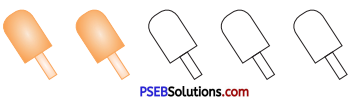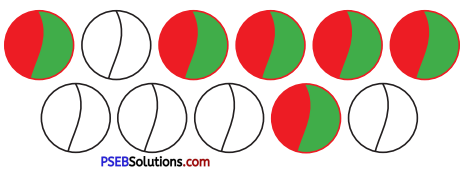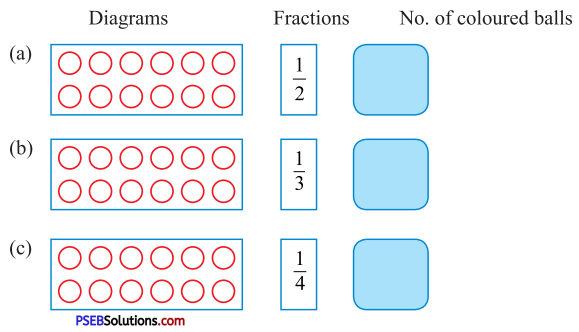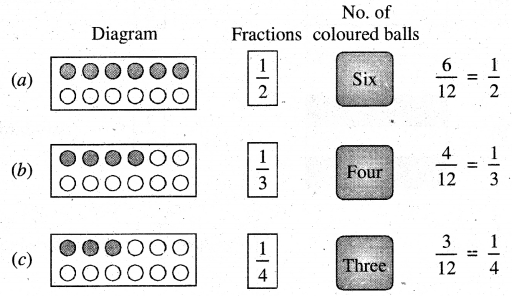# PSEB 5th Class Maths Solutions Chapter 4 Fractions Ex 4.1

Punjab State Board PSEB 5th Class Maths Book Solutions Chapter 4 Fractions Ex 4.1 Textbook Exercise Questions and Answers.

## PSEB Solutions for Class 5 Maths Chapter 4 Fractions Ex 4.1

Question 1.
Out of the following group of stars:(a) Write the fraction of coloured stars. ____
Solution:
$$\frac{4}{9}$$

(b) Write fraction of stars without colour. ____
Solution:
$$\frac{5}{9}$$

Question 2.
In the following diagram(a) Write fraction of coloured ice creams ____
Solution:
$$\frac{2}{5}$$

(b) Write fraction of ice creams without colour
Solution:
$$\frac{3}{5}$$Question 3.
In the following diagram:(a) Write fraction of coloured balls. ____
Solution:
$$\frac{6}{11}$$

(b) Write fraction of balls without colour.
Solution:
$$\frac{5}{11}$$

Question 4.
There are 12 balls in each of the following box. Colour the balls according to given fraction in the box and write number of coloured balls in blank box :Solution: